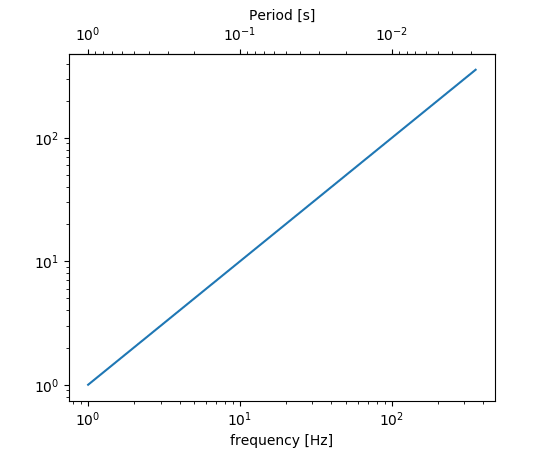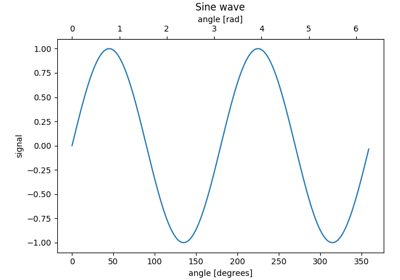/Matplotlib 3.1

# matplotlib.axes.Axes.secondary_xaxis

`Axes.secondary_xaxis(self, location, *, functions=None, **kwargs)` [source]

Add a second x-axis to this axes.

For example if we want to have a second scale for the data plotted on the xaxis.

Parameters: `location : string or scalar` The position to put the secondary axis. Strings can be 'top' or 'bottom', for x-oriented axises or 'left' or 'right' for y-oriented axises or a scalar can be a float indicating the relative position on the axes to put the new axes (0 being the bottom (left), and 1.0 being the top (right).) `functions : 2-tuple of func, or Transform with an inverse` If a 2-tuple of functions, the user specifies the transform function and its inverse. i.e. `functions=(lambda x: 2 / x, lambda x: 2 / x)` would be an reciprocal transform with a factor of 2. The user can also directly supply a subclass of `transforms.Transform` so long as it has an inverse. See Secondary Axis for examples of making these conversions. `ax : axes._secondary_axes.SecondaryAxis` `**kwargs : Axes properties.` Other miscellaneous axes parameters.

Warning

This method is experimental as of 3.1, and the API may change.

#### Examples

The main axis shows frequency, and the secondary axis shows period.## Examples using `matplotlib.axes.Axes.secondary_xaxis`Secondary Axis